International
Tables for
Crystallography
Volume B
Reciprocal space
Edited by U. Shmueli

International Tables for Crystallography (2006). Vol. B. ch. 2.5, pp. 322-323   | 1 | 2 |

## Section 2.5.7.3. Probabilistic estimate of phase invariant sums

D. L. Dorsete*

#### 2.5.7.3. Probabilistic estimate of phase invariant sums

| top | pdf |

Conventional direct phasing techniques, as commonly employed in X-ray crystallography (e.g. see Chapter 2.2), have also been used for ab initio electron-crystallographic analyses. As in X-ray crystallography, probabilistic estimates of a linear combination of phases (Hauptman & Karle, 1953; Hauptman, 1972) are made after normalized structure factors are calculated via electron form factors, i.e.(Here, an overall temperature factor can be found from a Wilson plot. Because of multiple scattering, the value of B may be found occasionally to lie close to 0.0 Å2.) The phase invariant sums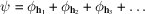can be particularly effective for structure analysis. Of particular importance historically have been the-triple invariants where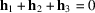and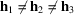. The probability of predictingis directly related to the value of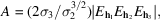where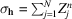and Z is the value of the scattering factor at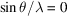. Thus, the values of the phases are related to the measured structure factors, just as they are found to be in X-ray crystallography. The normalization described above imposes the point-atom structure (compensating for the fall-off of an approximately Gaussian form factor) often assumed in deriving the joint probability distributions. Especially for van der Waals structures, the constraint of positivity also holds in electron crystallography. (It is also quite useful for charged atoms so long as the reflections are not measured at very low angles.) Other useful phase invariant sums are thetriples, where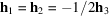, and the quartets, where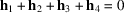and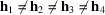. The prediction of a correct phase for an invariant is related in each case to the normalized structure-factor magnitudes.

The procedure for phase determination, therefore, is identical to the one used in X-ray crystallography (see Chapter 2.2). Using vectorial combinations of Miller indices, one generates triple and quartet invariants from available measured data and ranks them according to parameters such as A, defined above, which, as shown in Chapter 2.2, are arguments of the Cochran formula. The invariants are thus listed in order of their reliability. This, in fact, generates a set of simultaneous equations in crystallographic phase. In order to begin solving these equations, it is permissible to define arbitrarily the phase values of a limited number of reflections (three for a three-dimensional primitive unit cell) for reflections with Miller-index parity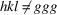and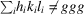, where g is an even number. This defines the origin of a unit cell. For noncentrosymmetric unit cells, the condition for defining the origin, which depends on the space group, is somewhat more complicated and an enantiomorph-defining reflection must be added.

In the evaluation of phase-invariant sums above a certain probability threshold, phase values are determined algebraically after origin (and enantiomorph) definition until a large enough set is obtained to permit calculation of an interpretable potential map (i.e. where atomic positions can be seen). There may be a few invariant phase sums above this threshold probability value which are incorrectly predicted, leading either to false phase assignments or at least to phase assignments inconsistent with those found from other invariants. A small number of such errors can generally be tolerated. Another problem arises when an insufficient quantity of new phase values is assigned directly from the phase invariants after the origin-defining phases are defined. This difficulty may occur for small data sets, for example. If this is the case, it is possible that a new reflection of proper index parity can be used to define the origin. Alternatively,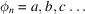algebraic unknowns can be used to establish the phase linkage among certain reflections. If the structure is centrosymmetric, and when enough reflections are given at least symbolic phase assignments,maps are calculated and the correct structure is identified by inspection of the potential maps. When all goes well in this so-called `symbolic addition' procedure, the symbols are uniquely determined and there is no need to calculate more than a single map. If algebraic values are retained for certain phases because of limited vectorial connections in the data set, then a few maps may need to be generated so that the correct structure can be identified using the chemical knowledge of the investigator. The atomic positions identified can then be used to calculate phases for all observed data (via the structure-factor calculation) and the structure can be refined by Fourier (or, sometimes, least-squares) techniques to minimize the crystallographic R factor.

The first actual application of direct phasing techniques to experimental electron-diffraction data, based on symbolic addition procedures, was to two methylene subcell structures (an n-paraffin and a phospholipid; Dorset & Hauptman, 1976). Since then, evaluation of phase invariants has led to numerous other structures. For example, early texture electron-diffraction data sets obtained in Moscow (Vainshtein, 1964) were shown to be suitable for direct analysis. The structure of diketopiperazine (Dorset, 1991a) was determined from these electron-diffraction data (Vainshtein, 1955) when directly determined phases allowed computation of potential maps such as the one shown in Fig. 2.5.7.1. Bond distances and angles are in good agreement with the X-ray structure, particularly after least-squares refinement (Dorset & McCourt, 1994a). In addition, the structures of urea (Dorset, 1991b), using data published by Lobachev & Vainshtein (1961), paraelectric thiourea (Dorset, 1991b), using data published by Dvoryankin & Vainshtein (1960), and three mineral structures (Dorset, 1992a), from data published by Zvyagin (1967), have been determined, all using the original texture (or mosaic single-crystal) diffraction data. The most recent determination based on such texture diffraction data is that of basic copper chloride (Voronova & Vainshtein, 1958; Dorset, 1994c).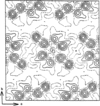Figure 2.5.7.1 | top | pdf |Potential map for diketopiperazine ( projection) after a direct phase determination with texture electron-diffraction intensity data obtained originally by Vainshtein (1955).

Symbolic addition has also been used to assign phases to selected-area diffraction data. The crystal structure of boric acid (Cowley, 1953) has been redetermined, adding an independent low-temperature analysis (Dorset, 1992b). Additionally, a direct structure analysis has been reported for graphite, based on high-voltage intensity data (Ogawa et al., 1994). Two-dimensional data from several polymer structures have also been analysed successfully (Dorset, 1992c) as have three-dimensional intensity data (Dorset, 1991c,d; Dorset & McCourt, 1993).

Phase information from electron micrographs has also been used to aid phase determination by symbolic addition. Examples include the epitaxically oriented paraffins n-hexatriacontane (Dorset & Zemlin, 1990), n-tritriacontane (Dorset & Zhang, 1991) and a 1:1 solid solution of n-C32H66/n-C36H74 (Dorset, 1990a). Similarly, lamellar electron-diffraction data to ca 3 Å resolution from epitaxically oriented phospholipids have been phased by analysis ofand-triplet invariants (Dorset, 1990b, 1991e,f), in one case combined with values from a 6 Å resolution electron microscope image (Dorset et al., 1990, 1993). Most recently, such data have been used to determine the layer packing of a phospholipid binary solid solution (Dorset, 1994d).

An ab initio direct phase analysis was carried out with zonal electron-diffraction data from copper perchlorophthalocyanine. Using intensities from a ca 100 Å thick sample collected at 1.2 MeV, the best map from a phase set with symbolic unknowns retrieves the positions of all the heavy atoms, equivalent to the results of the best images (Uyeda et al., 1978–1979). Using these positions to calculate an initial phase set, the positions of the remaining light C, N atoms were found by Fourier refinement so that the final bond distances and angles were in good agreement with those from X-ray structures of similar compounds (Dorset et al., 1991). A similar analysis has been carried out for the perbromo analogue (Dorset et al., 1992). Although dynamical scattering and secondary scattering significantly perturb the observed intensity data, the total molecular structure can be visualized after a Fourier refinement. Most recently, a three-dimensional structure determination was reported for C60 buckminsterfullerene based on symbolic addition with results most in accord with a rotationally disordered molecular packing (Dorset & McCourt, 1994b).

### ReferencesCowley, J. M. (1953). Structure analysis of single crystals by electron diffraction. II. Disordered boric acid structure. Acta Cryst. 6, 522–529.Google ScholarDorset, D. L. (1990a). Direct structure analysis of a paraffin solid solution. Proc. Natl Acad. Sci. USA, 87, 8541–8544.Google ScholarDorset, D. L. (1990b). Direct determination of crystallographic phases for diffraction data from phospholipid multilamellar arrays. Biophys. J. 58, 1077–1087.Google ScholarDorset, D. L. (1991a). Electron diffraction structure analysis of diketopiperazine – a direct phase determination. Acta Cryst. A47, 510–515.Google ScholarDorset, D. L. (1991b). Is electron crystallography possible? The direct determination of organic crystal structures. Ultramicroscopy, 38, 23–40.Google ScholarDorset, D. L. (1991c). Electron diffraction structure analysis of polyethylene. A direct phase determination. Macromolecules, 24, 1175–1178.Google ScholarDorset, D. L. (1991d). Electron crystallography of linear polymers: direct structure analysis of poly(-caprolactone). Proc. Natl Acad. Sci. USA, 88, 5499–5502.Google ScholarDorset, D. L. (1991e). Direct determination of crystallographic phases for diffraction data from lipid bilayers. I. Reliability and phase refinement. Biophys. J. 60, 1356–1365.Google ScholarDorset, D. L. (1991f). Direct determination of crystallographic phases for diffraction data from lipid bilayers. II. Refinement of phospholipid structures. Biophys. J. 60, 1366–1373.Google ScholarDorset, D. L. (1992a). Direct phasing in electron crystallography: determination of layer silicate structures. Ultramicroscopy, 45, 5–14.Google ScholarDorset, D. L. (1992b). Direct methods in electron crystallography – structure analysis of boric acid. Acta Cryst. A48, 568–574.Google ScholarDorset, D. L. (1992c). Electron crystallography of linear polymers: direct phase determination for zonal data sets. Macromolecules, 25, 4425–4430.Google ScholarDorset, D. L. (1994c). Electron crystallography of inorganic compounds. Direct determination of the basic copper chloride structure CuCl2·3Cu(OH)2. J. Chem. Crystallogr. 24, 219–224.Google ScholarDorset, D. L. (1994d). Direct determination of layer packing for a phospholipid solid solution at 0.32 nm resolution. Proc. Natl Acad. Sci. USA, 91, 4920–4924.Google ScholarDorset, D. L., Beckmann, E. & Zemlin, F. (1990). Direct determination of a phospholipid lamellar structure at 0.34 nm resolution. Proc. Natl Acad. Sci. USA, 87, 7570–7573.Google ScholarDorset, D. L. & Hauptman, H. A. (1976). Direct phase determination for quasi-kinematical electron diffraction intensity data from organic microcrystals. Ultramicroscopy, 1, 195–201.Google ScholarDorset, D. L. & McCourt, M. P. (1993). Electron crystallographic analysis of a polysaccharide structure – direct phase determination and model refinement for mannan I. J. Struct. Biol. 111, 118–124.Google ScholarDorset, D. L. & McCourt, M. P. (1994a). Automated structure analysis in electron crystallography: phase determination with the tangent formula and least-squares refinement. Acta Cryst. A50, 287–292.Google ScholarDorset, D. L. & McCourt, M. P. (1994b). Disorder and molecular packing of C60 buckminsterfullerene: a direct electron-crystallographic analysis. Acta Cryst. A50, 344–351.Google ScholarDorset, D. L., McCourt, M. P., Tivol, W. F. & Turner, J. N. (1993). Electron diffraction from phospholipids – an approximate correction for dynamical scattering and tests for a correct phase determination. J. Appl. Cryst. 26, 778–786.Google ScholarDorset, D. L., Tivol, W. F. & Turner, J. N. (1991). Electron crystallography at atomic resolution: ab initio structure analysis of copper perchlorophthalocyanine. Ultramicroscopy, 38, 41–45.Google ScholarDorset, D. L., Tivol, W. F. & Turner, J. N. (1992). Dynamical scattering and electron crystallography – ab initio structure analysis of copper perbromophthalocyanine. Acta Cryst. A48, 562–568.Google ScholarDorset, D. L. & Zemlin, F. (1990). Direct phase determination in electron crystallography: the crystal structure of an n-paraffin. Ultramicroscopy, 33, 227–236.Google ScholarDorset, D. L. & Zhang, W. P. (1991). Electron crystallography at atomic resolution: the structure of the odd-chain paraffin n-tritriacontane. J. Electron Microsc. Tech. 18, 142–147.Google ScholarDvoryankin, V. F. & Vainshtein, B. K. (1960). An electron diffraction study of thiourea. Sov. Phys. Crystallogr. 5, 564–574.Google ScholarHauptman, H. (1972). Crystal structure determination. The role of the cosine seminvariants. NY: Plenum Press.Google ScholarHauptman, H. & Karle, J. (1953). Solution of the phase problem. I. The centrosymmetric crystal. American Crystallographic Association Monograph No. 3. Ann Arbor, MI: Edwards Brothers.Google ScholarLobachev, A. N. & Vainshtein, B. K. (1961). An electron diffraction study of urea. Sov. Phys. Crystallogr. 6, 313–317.Google ScholarOgawa, T., Moriguchi, S., Isoda, S. & Kobayashi, T. (1994). Application of an imaging plate to electron crystallography at atomic resolution. Polymer, 35, 1132–1136.Google ScholarUyeda, N., Kobayashi, T., Ishizuka, K. & Fujiyoshi, Y. (1978–1979). High voltage electron microscopy for image discrimination of constituent atoms in crystals and molecules. Chem. Scr. 14, 47–61.Google ScholarVainshtein, B. K. (1955). Elektronograficheskoe issledovanie diketopiperazina. Zh. Fiz. Khim. 29, 327–344.Google ScholarVainshtein, B. K. (1964). Structure analysis by electron diffraction. Oxford: Pergamon Press.Google ScholarVoronova, A. A. & Vainshtein, B. K. (1958). An electron diffraction study of CuCl2·3Cu(OH)2. Sov. Phys. Crystallogr. 3, 445–451.Google ScholarZvyagin, B. B. (1967). Electron-diffraction analysis of clay mineral structures. New York: Plenum.Google Scholar